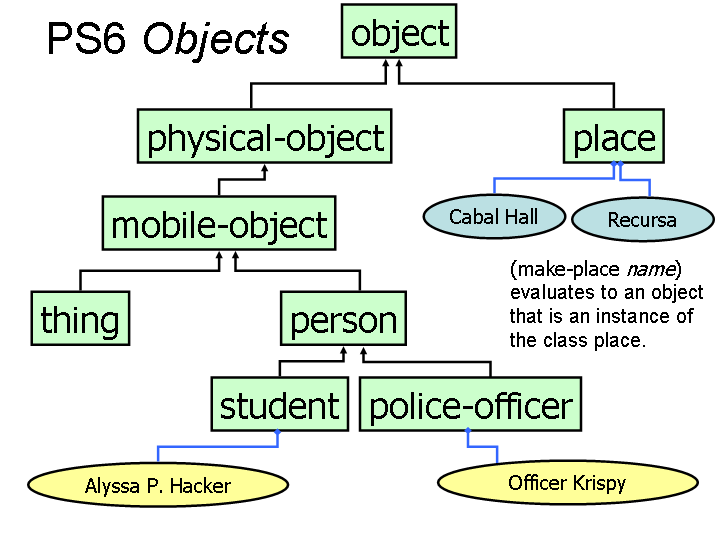University of Virginia, Department of Computer Science CS200: Computer Science, Spring 2004

Notes: Wednesday 17 March 2004

• Since we will not cover everything you need to know for PS6 in class until Monday, you now have two options for Problem Set 6:
• Turn it in on Monday, 22 March as originally scheduled, but without answering questions 7 and 8.
• Turn it in on Monday, 29 March (one week later, answering all questions).
• Monday, 22 March: Read GEB, Aria with Diverse Variations and Chapter 13. This chapter proves that the halting problem is not decidable, and introduces the Church-Turing Thesis (which we will explore more in later classes). You will not be assigned to read Chapter XIV, but it goes into more depth on Gödel's proof and is recommended.
• Problem Set 7 will be out on 29 March and due on 7 April.
• Problem Set 8 will be out on 7 April; part I will be due on 12 April, part II will be due on 26 April (last day of class).
• Exam 2 will be handed out on 14 April and due on 19 April.
Notes
```(define (make-number n)
(lambda (message)
(cond
((eq? message 'value) (lambda (self) n))
((eq? message 'add) (lambda (self other)

(define (ask object message . args) (apply (object message) object args))
```
Inheritance is using the definition of one class to make another class.
```(define (make-fraction numer denom)
(let ((super (make-number #f)))
(lambda (message)
(cond
((eq? message 'value) (lambda (self) (/ numer denom)))
((eq? message 'get-denominator) (lambda (self) denom))
((eq? message 'get-numerator) (lambda (self) numer))
(else (super message))))))
```
The class fraction inherits from number.
fraction is a subclass of number.
The superclass of fraction is number.

Which of the traced applications are from fraction and which are from number?
```> (ask half 'add half)
| #f
| #f
| #f
| #f
| #t
| |(eq? value value)
| |#t
| 1/2
| |(eq? value value)
| |#t
| 1/2
|1
1
```If I have seen further it is by standing on the shoulders of giants.
Isaac Newton (on inheritance?)

If I have not seen as far as others, it is because giants were standing on my shoulders.
Hal Abelson (on subtyping?)